#### 2018

Measurement-induced nonlinear transformations — We considered the task of deciding whether an unknown qubit state falls in a prescribed neighborhood of a reference state. If several copies of the unknown state are given and we can apply a unitary operation pairwise on them combined with a postselection scheme conditioned on the measurement result obtained on one of the qubits of the pair, the resulting transformation is a deterministic, nonlinear, chaotic map in the Hilbert space. We derived a class of these transformations capable of orthogonalizing nonorthogonal qubit states after a few iterations. These nonlinear maps orthogonalize states which correspond to the two different convergence regions of the nonlinear map. Based on the analysis of the border (the so-called Julia set) between the two regions of convergence, we showed that it is always possible to find a map capable of deciding whether an unknown state is within a neighborhood of fixed radius around a desired quantum state. We analyzed which one- and two-qubit operations would physically realize the scheme. It is possible to find a single two-qubit unitary gate for each map or, alternatively, a universal special two-qubit gate together with single-qubit gates in order to carry out the task. We note that it is enough to have a single physical realization of the required gates due to the iterative nature of the scheme.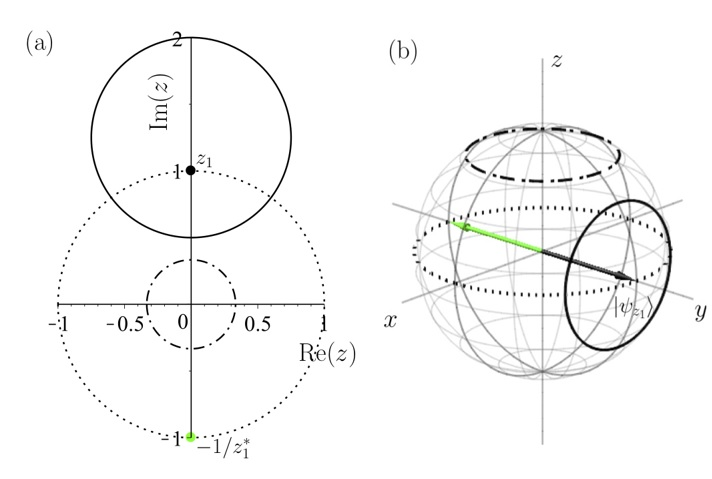Figure 1. The decomposition of the relevant map f into the subsequent actions of a contracting two-qubit operation and a single-qubit unitary operation. (a) The effect of the decomposition on the complex plane (projection of the Bloch sphere), representing the initial states of the qubit. States within the solid circle are matched. (b) The same decomposition represented on the Bloch sphere.

Quantum walks — Measurements on a quantum particle unavoidably affect its state, since the otherwise unitary evolution of the system is interrupted by a nonunitary projection operation. To probe measurement-induced effects in the state dynamics using a quantum simulator, the challenge is to implement controlled measurements on a small subspace of the system and continue the evolution from the complementary subspace. A powerful platform for versatile quantum evolution is represented by photonic quantum walks because of their high control over all relevant parameters. However, measurement-induced dynamics in such a platform have not yet been realized. We participated in the implementation (at the University of Paderborn) of controlled measurements in a discrete-time quantum walk based on time-multiplexing. This was achieved by adding a deterministic outcoupling of the optical signal to include measurements constrained to specific positions resulting in the projection of the walker’s state on the remaining ones. With this platform and coherent input light, we experimentally simulated measurement-induced single-particle quantum dynamics. We demonstrated the difference between dynamics with only a single measurement at the final step and those including measurements during the evolution. To this aim, we studied recurrence as a figure of merit, that is, the return probability to the walker’s starting position, which was measured in the two cases. We tracked the development of the return probability over 36 time steps and observed the onset of both recurrent and transient evolution as an effect of the different measurement schemes, a signature which only emerges for quantum systems. Our simulation of the observed one-particle conditional quantum dynamics does not require a genuine quantum particle but was demonstrated with coherent light.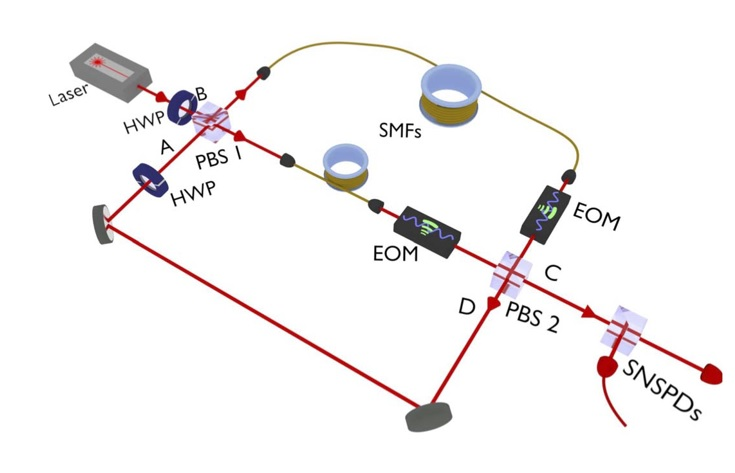Figure 2. Schematic of the experimental setup of the time-multiplexed quantum walk with active in- and outcoupling realized by two EOMs (electro-optical modulators). The active control of the switches allows to implement in the time domain both the continual and reset measurement schemes. HWP, half-wave plate; PBS, polarizing beam splitter; SMF, single-mode fiber; SNSPDs, superconducting nanowire single-photon detectors.

Ro-vibrational quantum states in molecules — We determined the internal-axis system (IAS) of molecules with a large amplitude internal motion (LAM) by integrating the kinematic equation of the IAS by Lie-group and Lie-algebraic methods. Numerical examples on hydrogen peroxide, nitrous acid, and acetaldehyde demonstrate the methods. By exploiting the special product structure of the solution matrix, we devised simple methods for calculating the transformation to the rho-axis system (RAS) along with the value of the parameter ρ characterizing a RAS rotational-LAM kinetic energy operator. The parameter ρ so calculated agrees exactly with that one obtained by the Floquet method as shown in the example of acetaldehyde. We gave geometrical interpretation of ρ. We numerically demonstated the advantageous property of the RAS over the IAS in retaining simple periodic boundary conditions.

Nanophotonics — Nonlinear second harmonic wave generation (SHG) has been thoroughly examined in one dimension both analytically and numerically. Recently, the application of advanced domain poling techniques enabled the fabrication of two-dimensional (2D) patterns of the sign of the nonlinear coefficient in certain nonlinear crystals, such as LiNbO3 and LiTaO3 . This method can be used to achieve quasi phase matching in SHG and hence amplification of the second harmonic fields in 2D. We have worked out a true vectorial numerical method for the simulation of SHG by extending the finite difference frequency domain method (FDFD). Our nonlinear method (NL-FDFD) operates directly on the electromagnetic fields, uses two meshes for the simulation (for ω and 2ω fields), and handles the nonlinear coupling as an interaction between the two meshes. Final field distributions can be obtained by a small number of iteration steps. NL-FDFD can be applied in arbitrarily structured linear media with an arbitrarily structured χ^(2) component both in the small conversion efficiency and the pump depleted cases.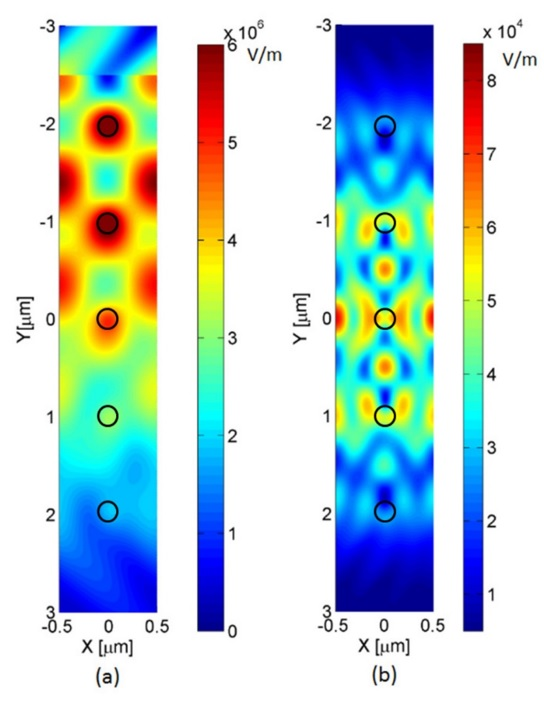Figure 3. Magnitude of the Ez field components for  (a) fundamental wave (b) second harmonic wave at the  resonant frequency for five arrays of cylinders. We show here the result of a model calculation: the underlying dielectric structure consists of periodic arrays of nonlinear cylinders, it is infinite in the x-direction and there are five periods in the y-direction. The fundamental wave propagates in the positive y-direction, and it can be tilted from normal incidence. At certain angles and frequencies the structure exhibits double resonance: the reflectivity is close to unity both for the fundamental and for the second harmonic wave

#### 2017

Measurement-induced non-linear transformations. — We proposed a cavity quantum electrodynamical scenario for implementing a Schrödinger microscope capable of amplifying differences between non-orthogonal atomic quantum states. The scheme involves an ensemble of identically prepared two-level atoms interacting pairwise with a single mode of the radiation field as described by the Tavis-Cummings model (Fig. 1).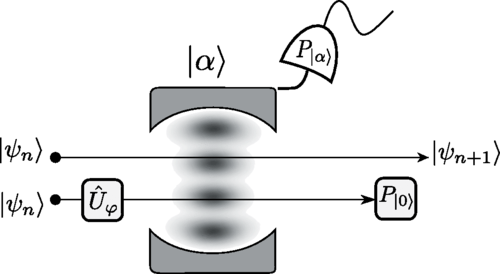Figure 1. Illustration of the scheme. Two two-level atoms in the same state interact with the cavity field prepared in a coherent state. Before the interaction, a unitary gate is applied to one of the atoms, and after the interaction and the projection of the field onto the initial coherent state, this same atom is projected onto its ground state. Finally, the other atom is left in a non-linearly transformed state.

We showed that by repeated measurements of the cavity field and of one atom within each pair, a measurement-induced non-linear quantum transformation of the relevant atomic states can be realized. The intricate dynamical properties of this non-linear quantum transformation, which exhibits measurement-induced chaos, allow approximate orthogonalization of atomic states by purification after a few iterations of the protocol and, thus, the application of the scheme for quantum state discrimination.

Topological phases. — We discovered topological features of the Hofstadter butterfly spectra of periodically driven systems. The butterfly is the fractal spectrum of energy eigenstates of a quantum lattice system in a magnetic field. It was discovered numerically (1976, predating the word "fractal"), analyzed analytically (contributing to the topological understanding of the quantum Hall effect), and it is about to be observed experimentally on laser-trapped cold atoms and in graphene. We found that in periodically driven systems, where the drive is very far from just a perturbation, the Hofstadter butterfly can "take flight", i.e., can "wind" in quasienergy (Fig. 2). This behaviour is closely related to a recently discovered topological invariant unique to such non-perturbatively driven systems, and gives us a way to numerically evaluate and perhaps experimentally observe this invariant in an efficient way.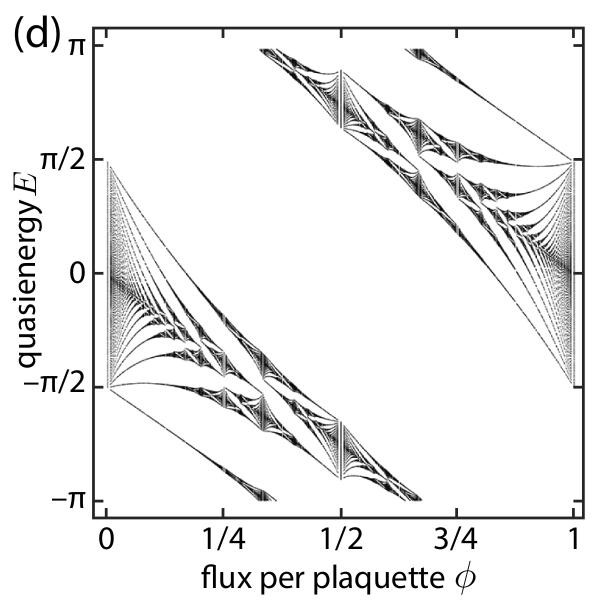Figure 2. The spectrum of quasienergies of a periodically driven system (quantum walk) can wind as a function of applied magnetic field. The winding is quantized, and reveals a bulk topological invariant of the system.

Ro-vibrational quantum states in molecules. — Recently, a general expression for Eckart-frame Hamilton operators has been obtained by the gateway Hamiltonian method. The kinetic energy operator in this general Hamiltonian is nearly identical to that of the Eckart-Watson operator even when curvilinear vibrational coordinates are employed. Its different realizations correspond to different methods of calculating Eckart displacements. There are at least two different methods for calculating such displacements: rotation and projection. In our work, the application of Eckart Hamiltonian operators constructed by rotation and projection was numerically demonstrated in calculating vibrational energy levels. The numerical examples confirm that there is no need for rotation to construct an Eckart ro-vibrational Hamiltonian. The application of the gateway method is advantageous even when rotation is used since it obviates the need for differentiation of the matrix rotating into the Eckart frame. Simple geometrical arguments explain that there are infinitely many different methods for calculating Eckart displacements. The geometrical picture also suggests that a unique Eckart displacement vector may be defined as the shortest (mass-weighted) Eckart displacement vector among Eckart displacement vectors corresponding to configurations related by rotation. Its length, as shown analytically and demonstrated by numerical examples, is equal to or less than that of the Eckart displacement vector one can obtain by rotation to the Eckart frame.

Nanophotonics. — We have worked out a true vectorial numerical method for the simulation of the non-linear second harmonic generation process by extending the finite difference frequency domain method (FDFD). Our non-linear method (NL-FDFD) operates directly on the electromagnetic fields, uses two meshes for the simulation (for ω and 2ω fields) and handles the non-linear coupling as an interaction between the two meshes. Final field distributions can be obtained by a small number of iteration steps. NL-FDFD can be applied in arbitrarily structured linear media with an arbitrarily structured χ (2) component both in the small-conversion-efficiency and the pump-depleted cases.